# A/B testing 5个问题

Microsoft在KDD2007时的paper《Practical Guide to Controlled Experiments on the Web:Listen to Your Customers not to the HiPPO》中，对A/B testing系统设计有一些practical guide，可以参考一下：

# 3.controlled experiments

## 3.1 术语

• OEC
• Factor
• Variant
• Experimentation Unit
• Null Hypothesis
• Confidence level
• Power
• A/A Test
• Standard Deviation (Std-Dev)
• Standard Error (Std-Err)

## 3.2 Hypothesis Testing和Sample Size

• 置信等级（confidence level）。通常设置为95%，该level表示：5%的机会是不正确的
• 统计功效（Power）。通常在80-95%间，尽管不是直接控制的。如果零假设是false的（比如：在OEC上有差异）, power表示决定该差异的概率，是统计显著的。（Type II error）
• (Standard Error)。Std-Err越小，test越强。有三种方法减小std-err：
• 被估计的OEC通常是大样本的一个平均。如3.1所示，一个均值(mean)的Std-Err会随着sample size的均方根按比例递减，因此，增大sample size（这通常意味着运行实验更久），可以减小Std-Err，从而增大power
• 使用天然具有更小变动性的OEC components（例如：Std-Dev, $\delta$），std-err会越小. 例如，转化率（conversion probability 0-100%）通常比购买单元数目（通常是小整数）具有更低的Std-Dev，换句话说，它比收益（revenue: real-valued）具有一个更低的std-dev。
• 通过过滤掉那些没有曝光给variants的用户，OEC的可变性会越低. 例如，如果你对checkout page做出一个变更，只分析访问该page的用户，由于每个人都增加了噪声(noise)，会增加可变性（variability）
• 4.the effect：对于variants来说在OECs上的差异。差异越大越容易检测，因此，great ideas不可能错过。相反的，如果Type I或Type II errors发生，当该effects很小时，他们更可能发生。

$n = (4r\delta / \Delta)^2$

• n是sample size
• r是variants数目
• $\delta$是OEC的std-dev
• $\Delta$是OECs之间的最小差异
• 4是因子，表示对于大的n会估计过高25%，但该近似会满足下面示例。

## 3.3 online settings的扩展

basic controlled experiments的一些扩展在online setting中是可能的。

## 3.4 限制

• 2.短期效应(short term) vs. 长期效应（long term effects）。controlled experiments会在实验期间（通常数周）衡量OEC的effect。而一些paper作者则批评说：这样只会注某一指标意味着short-term focus，我们对此不赞同。long-term目标应该是OEC的一部分。假设我们以搜索广告为例。如果你的OEC是收益（revenue），你可能在一个页面上粘帖数个广告，但我们知道：越多广告会伤害用户体验，因此一个好的OEC应包含对于没有点击的房地产广告的一个惩项（penalty term），或／和 对重复访问和放弃的直接measure。同样的，查看一个延迟的转化指标是明智的，其中从一个用户被曝光某样东西到该用户做出动作这段时间内有个迟延(lag)。这有时被称为潜在转化（latent conversions）。找好好的OEC是很难的，但有什么替代方法吗？这里的关键是，认识到该限制。
• 3.首效应(Primacy effects)和新效应（newness effects）。有一些负面效应需要认识。如果你在一个web网站上更换了导航，体验的用户可能更低效，直到他们完全适应了新导航，因而Control组会天然具有优势。相反的，如果一个新设计或新特性被引入，一些用户可能会研究它，到处进行点击，从而引入一个“newness” bias。该bias有时会与霍索恩效应（Hawthorne effect）相关。Primacy和Newness这两者意味着：一些实验需要运行多周。可以做个分析：为不同variants上的新用户计算OEC，因为他们不会受两个因素影响。
• 4.必须实现的特性。一个真实的controlled experiment需要曝光一些用户给一个Treatment，不同于当前站点（Control组）。该prototype可能是个prototype，它可以在很小比例上进行测试，或者不会覆盖所有的边缘情况（edge cases）（例如：实验可能故意排除20%的需要显著测试的浏览器类型）。尽管如些，该特性必须被实现，并曝光给用户足够的量。Jacob(34)正确指出：paper prototyping可以被用于在早期定性反馈和快速改进设计。我们赞同并推荐这样的技术来完善controlled experiments。
• 6.并行实验（Parallel Experiments）。我们的实验实际上很少发生很强的交叉（33），我们认为这一点是过度关注了。提升对这一点的关注对于实验者来说足够避免交叉（interact）的测试。成对的统计测试（statistical test）也可以完成，来自动标记这样的交叉（interactions）。
• 7.启动事件和媒体公告。如果对新特性做出一个大的公告，（比如：该特性通过媒体进行公告），所有用户需要看到它。

# 4.多因子实验(Multi-Variable Testing)

• 1.你可以在一个较短时间内测试许多factors，加速提升。例如，你想测试在网站上的5个变化，你需要每个A/B test运行4周时间来达到你需要的power，它会至少需要5个月来完成A/B tests。然而，你可以使用所有5个factors来运行单个MVT，它只需要1个月就可以达到5个A/B tests相同的power。
• 2.你可以估计factors间的交叉。两个factors的交叉，如果它们的组合效应与两个单独的effects叠加不同。如果这两个factors一起可以增强结果，那么该交叉（interaction）是协同的（synergistic）。如果相反的，他们相互抵触会抑制该效果，该交叉（interaction）是对抗的（antagonistic）。

• 1.一些factors的组合可能会给出一个较差的用户体验。例如，对于一个在线零售商来说，正在测试的两个factors可能是：增加一个商品的图片 or 提供额外的商品详情。当单独进行测试时，两者分别均会提升销售，但当两者在同时完成时，“buy box”会被推到折叠（fold）下面，这会减小销售。这就是一个大的对抗交叉（antagonistic interaction）。该交叉在计划阶段（planning phase）可以被捕获，以便这两个factors不会被同时测试。
• 2.分析和解释是更困难的。对于单个factor的test，你通常具有许多指标来进行Treatment-Control的比较。对于一个MVT，你需要为多个Treatment-Control比较设置相同的matrics（对于每个测试的factor至少要有一个），以及在factors间交叉的分析和解释。确定的是，该信息集越丰富，对于treatments的评估也越复杂。
• 3.它会花费更长时间开始测试。如果你具有希望测试的5个factors，并计划以一次测一个的方式测试它们，你可以启动任意待测的，接着测试其它的。使用一个MVT，你必须在test开始之前，具备所有5个准备好的testing。如果任何一个延误了，会延误test的启动。

# 5.实验架构

## 5.1 随机算法

• 1.用户必须等可能地看到一个实验的每个variant（假设：50-50 split）。对于任意指定variant必须是无偏的(no bias)。
• 2.对于单个user重复分配（repeat assignments）必须是一致的（consistent）；在对该网站的每次后续访问上，该user必须被分配到相同的variant上。
• 3.当多个实验同时并行运行时，实验之间必须没有关联（correlation）。在一个实验中，一个user被分配（assignment）到一个variant，对于被分配给其它任意实验的一个variant，在概率上没有影响
• 4.该算法必须支持单调递增（monotonic ramp-up），这意味着，如果没有对那些已经被配给Teatment的用户分配进行变更，用户看到一个Treatment的百分比可能会缓慢增加。

## 5.2 Assignment方法

Assignment方法是软件的一部分，它允许正实验的网站对于不同的用户执行不同的代码路径。有许多方法来实验一个assignment方法，优点和缺点各不同。

# 6.学到的课程

• (i) 分析
• (ii) trust & execution

# 2.相关工作

## 2.3 上下文个性化

• (a) Liu提出了 a social-aided context-aware recommender systems for books and movies. 会利用丰富的上下文信息来将user-item矩阵划分为多个矩阵
• (b) Jamali等人提出了: a context-dependent matrix factorization model to create user profiles for recommendation in social network 。

## 2.4 行为因子分解

“如果你将知觉（perception）看成是一种联系（contact）和洽谈(communion)的方式，那么，支配感知就是支配联系，限制和管理感知就是限制和管理联系。”—Erving Goffman, The Presentation of Self in Everyday Lif.

## 3.2 衡量行为间的不同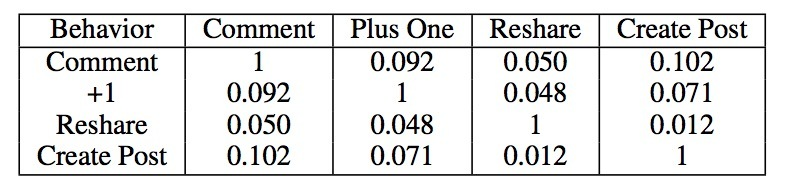# 4.问题定义

## 4.1 输入行为信号

$I = \lbrace t_i = (u_i, b_i, E_i), i=1, ..., N \rbrace$

• 每个$t_i$表示一个用户在社交媒体内容的某个特定片段上的动作。例如，一个$t_i$可以是：创建一个post，或者对某个post进行comment。
• $E_i$是该post的特征集合。

## 4.2 User profiles

$B = \lbrace P_u = \lbrace V_{u_B} \rbrace \rbrace$

• $P_u$是用户u的user profile，
• $V_{u_B}$是用户u在对应于她的行为类型B上的偏好向量。

$P_u$可以被认为是一个user tensor。

B即可以是单个行为类型（例如：创建一个post），或是一个不同行为类型的组合（例如：创建一个post和reshare一个post组合）。准确表述为：

$V_{u_B} = ( p_{u_B}^{e_1}, p_{u_B}^{e_2}, ..., p_{u_B}^{e_k} ), e_j \in E$

# 5.我们的方法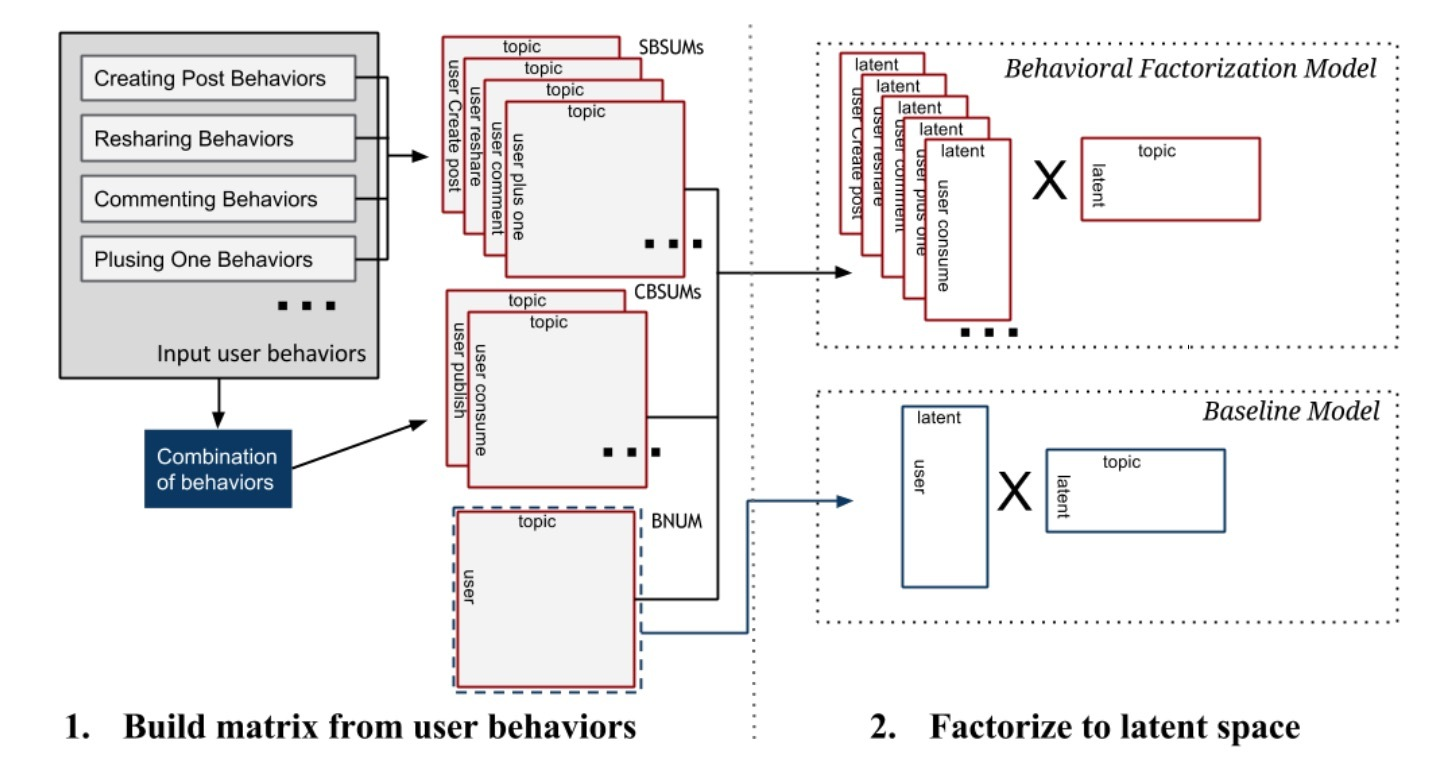• step 1: 给定第4节中定义的input user action tuples $I$，我们首先构建不同行为类型的矩阵。这对应于图1中的左部分。
• step 2: 我们对step 1中生成的矩阵进行因子分解来学到latent embedding space。这对应于图1中的右部分。
• step 3: 最后，我们使用学到的latent space来对兴趣主题做预测来构建user profiles。这会为每个用户u创建profiles $P_u = \lbrace V_{u_B} \rbrace$。这对应于图2.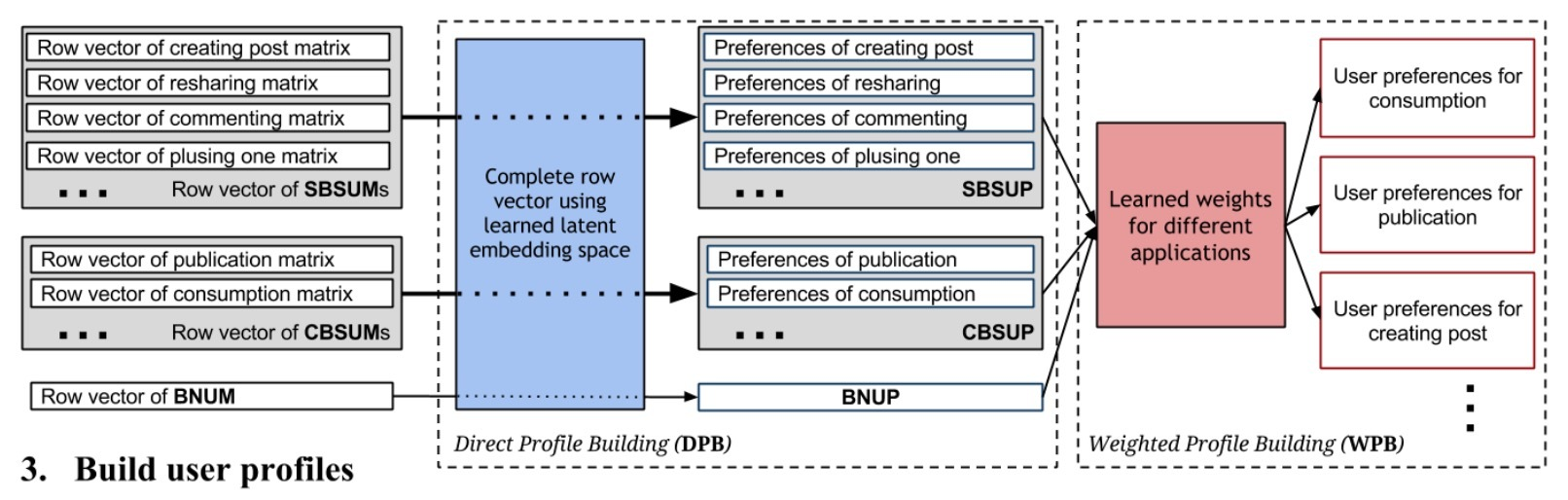## 5.1 step 1: 为不同行为类型构建矩阵

• (a) 传统的$N \times K$矩阵，被称为Behavior Non-specific User-topic Matrix(BNUM)
• (b) Single Behavior-Specific User-topic Matrix(SBSUM)
• (c) Combined Behavior-Specific User-topic Matrix(CBSUM)

### 5.1.1 BNUM (Behavior Non-specific User-topic Matrix)

$I_u = \lbrace t_j = (u_j, b_j, E_j) \rbrace, t_j \in I \wedge u_j = u$

$r_{ui} = r(I_u, i)$

$r_{ui} = \frac{(\sum\limits_{I_u} \sum\limits_{e \in E_j} \sigma_i(e)) + 1}{(\sum\limits_{I_u} \| E_j \|) + (\|\cup_{I_u} E_j\|)}$

…(1)

### 5.1.2 SBSUM (Single Behavior-Specific User-topic Matrix SBSUM)

SBSUM和CBSUM将行为类型单独划分来生成独立的user-topic矩阵。给定一个行为类型的特征集合$B \subset B$，我们想构建矩阵$R_B = \lbrace r_{ui}^B \rbrace$，其中每个条目表示从B中行为类型得到的隐式兴趣。

$r_{ui}^B = \frac{(\sum\limits_{I_u \wedge b_j \in B} \sum\limits_{e \in E_j} \sigma_i(e)) + 1}{ \sum\limits_{I_u \wedge b_j \in B} \| E_j \| ) + (\|\cup_{I_u \wedge b_j \in B} E_j \|)}$

…(2)

$\begin{cases} R_{b_1} = \lbrace r_{ui}^B \rbrace, B=\lbrace b_1,b_1 \in \mathscr{B} \rbrace \\ R_{b_2} = \lbrace r_{ui}^B \rbrace, B=\lbrace b_2,b_2 \in \mathscr{B} \rbrace \\ ... \\ R_{b_M} = \lbrace r_{ui}^B \rbrace, B=\lbrace b_M,b_M \in \mathscr{B} \rbrace \end{cases}$

…(3)

### 5.1.3 CBSUM（Combined Behavior Specific User-topic Matrix）

$\begin{cases} R_{B_1} = \lbrace r_{ui}^B \rbrace, B=\lbrace B_1,B_1 \in \mathscr{B} \rbrace \\ R_{B_2} = \lbrace r_{ui}^B \rbrace, B=\lbrace B_2,B_2 \in \mathscr{B} \rbrace \\ ... \\ R_{B_M} = \lbrace r_{ui}^B \rbrace, B=\lbrace b_M,b_M \in \mathscr{B} \rbrace \end{cases}$

…(4)

## 5.2 step 2: 学习latent embedding space

### 5.2.1 baseline: MF

$p_{ui} = \begin{cases} 1 , r_{ui}>0 \\ 0 , r_{ui}=0 \end{cases}$

$\min\limits_{x_*,y_*} \sum\limits_{ui} c_{ui} (p_{ui} - x_u^T y_i) ^ 2 + \lambda( \sum\limits_{u} \| x_u \|^2 + \sum\limits_i \| y_i \|^2)$

…(5)

### 5.2.2 行为因子分解模型（BF）

$min_{x_*,y_*} \sum\limits_{B \in \Gamma} \sum\limits_{u,i} c_{ui}^B (p_{ui}^B - x_u^{B^T} y_i)^2 + \lambda (\sum\limits_{B \in \Gamma} \sum\limits_{u} \| x_u^B \|^2 + \sum\limits_{i} \|y_i\|^2)$

….(6)

## 5.3 step 3: 构建user profiles

• i) 从profile矩阵的input row vectors构建direct profile
• ii) 通过一个回归模型学到一个权重集合，合并不同的direct profile进行构建weighted profile

• BNUP:
• SBSUP:
• CBSUP:

### 5.3.1 DPB(Direct Profile Building)

$P_u = \lbrace V_{u_B} = x_u^{B^T} Y \brace, B \in \Gamma$

…(7)

$V_{u_B} = ( p_{u_B}^{e_1}, p_{u_B}^{e_2}, ..., p_{u_B}^{e_K}), p_{u_B}^{e_i} = x_u^{B^T} y_1$

…(8)

$V_u = (p_u^{e_1}, p_u^{e_2}, ..., p_u^{e_K}), p_u^{e_i} = x_u^T y_i$

…(9)

### 5.3.2 WPB (Weighted Profile Building)

DPB会为一个用户生成一个behavior profile（如果该用户在过往有行为）。通过将用户的行为类型相分隔，我们可以使用DPB来为用户u的行为B生成profile，但这需要用户u在行为B上具有非零的观察值。对于那些在B上没有行为的用户，$V_{u_B}$会为空，这意味着一个没有该行为类型动作的user，不会有一个user profile。这在某种程度上对应于在推荐系统中的冷启动问题。

$V_{u_B} = \sum\limits_{B_t \in \Gamma} W_{B_t} x_u^{B_t^T} Y$

…(10)

$\Gamma$中不同行为类型的权重是模型级别的参数，例如：我们会为整个数据集的每个$B_t \in \Gamma$学到一个权重。因此，这些权重可以使用一个监督学习方法来从在我们的数据集中具有多种行为类型的所有用户上学到。因此，对于那些在过程历史记录中没有$B_t$的用户，我们仍可以为他们构建profiles。

# 6.评估

• H1: 从我们的行为因子分解方法中学到的latent embedding模型在构建user profiles比baseline MF模型要更好
• H2: 通过从多种行为类型中组合偏好向量，我们可以提升在特定行为类型上的user profiles的覆盖度(coverage)

## 6.1 实验设置

### 6.1.1 Dataset

• SBSUM
• Publication CBSUM
• Consumption CBSUM
• BNUM

### 6.1.2 评估指标

• Recall@N: $L_{method}$的top N的主题有多少出现在$L_{observed}$
• NDCG@N:
• AP@N:

# 2.二元离散化

$Ent(S) = - \sum_{i=1}^{k} P(C_i,S) log(P(C_i,S))$

$E(A,T;S) = \frac{|S_1|}{|S|} Ent(S_1) + \frac{|S_2|}{|S|} Ent(S_2)$

…(2)

A的二分离散化通过选择分割点$T_A$来决定，其中$E(A, T_A; S)$是所有候选分割点中的最小值。

## 2.1 分割点选择的讨论# 3.泛化该算法## 3.1 分割还是不分割？

$B = c_{11} Prob(d_A \wedge HT) + c_{22} Prob (d_R \wedge NT) + c_{12} Prob(d_A \wedge NT) + c_{21} Prob (d_R \wedge HT)$

## 3.2 MDLP(最小描述长度原则)

MDLP原则不必要要求与之前讨论的决策原则不同。它可以轻易地展示MDLP和Bayesian risk minimization strategy在理论上是相互相关的。由于篇幅原因，我们忽略了派生版本，它包含着可以包含对数据集S的hypothesis H所需要指定的bits数：$-log_2 (Prob(H|S))$，使用Bayes’ rule。最终获得的表达式等价于MDLP。这可以看成是采用MDLP的动机。

## 3.3 应用MDLP：编码问题

$log_2(N-1) + | S_1 | \cdot l_1 + | S_2 | \cdot l_2$ (bits)

$G_k = [ \sum_{k_1=1}^{k-1} (C_{k_1}^{k}) 2^{k_1}] + 2^k - 1 = 3^k - 2$

##

# 介绍

《BPR: Bayesian Personalized Ranking from Implicit Feedback》讨论了个性化排序学习模型的一个通用方法：Bayesian Personalized Ranking。主要贡献有：

• 1.描述了通用的优化方法：BPR-OPT，它来自于对最优个性化排序的最大后验估计。我们展示了BPR-OPT在AUC上的分析。
• 2.对于最大化BPR-OPT，我们提出了通用学习算法LearnBPR，它基于SGD，在训练过程使用bootstrap sampling。我们展示了该算法会优于最优化BPR-OPT时的SGD。
• 3.我们展示了如何应用learnBPR到两个state-of-art推荐模型中
• 4.我们的实验经验上展示了个性化排序任务，使用BPR的模型效果要好于其它学习算法

。。。

# 3.个性化排序（Personalized Ranking）

## 3.1 公式化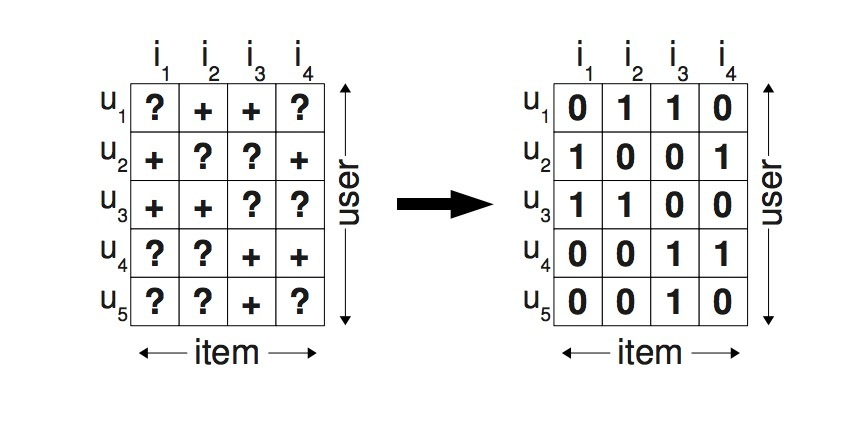\begin{aligned} & \forall i,j \in I: i \neq j \Rightarrow i >_u j \vee j >_u i \ \ (totality) \\ & \forall i,j \in I: i >_u j \wedge j >_u i \Rightarrow i = j \ \ (antisymmetry) \\ & \forall i,j \in I: i >_u j \wedge j >_u k \Rightarrow i >_u k \ \ (transitivity) \end{aligned}
• totallity: 总体性
• antisymmetry: 反对称性
• transitivity: 传递性

$I_u^+ := {i \in I: (u,i) \in S} \\ U_i^+ := {u \in U: (u,i) \in S}$

## 3.2 问题分析

• 正例：$(u, i) \in S$ pairs
• 负例：所有在$(U \times I) \backslash S$中的其它组合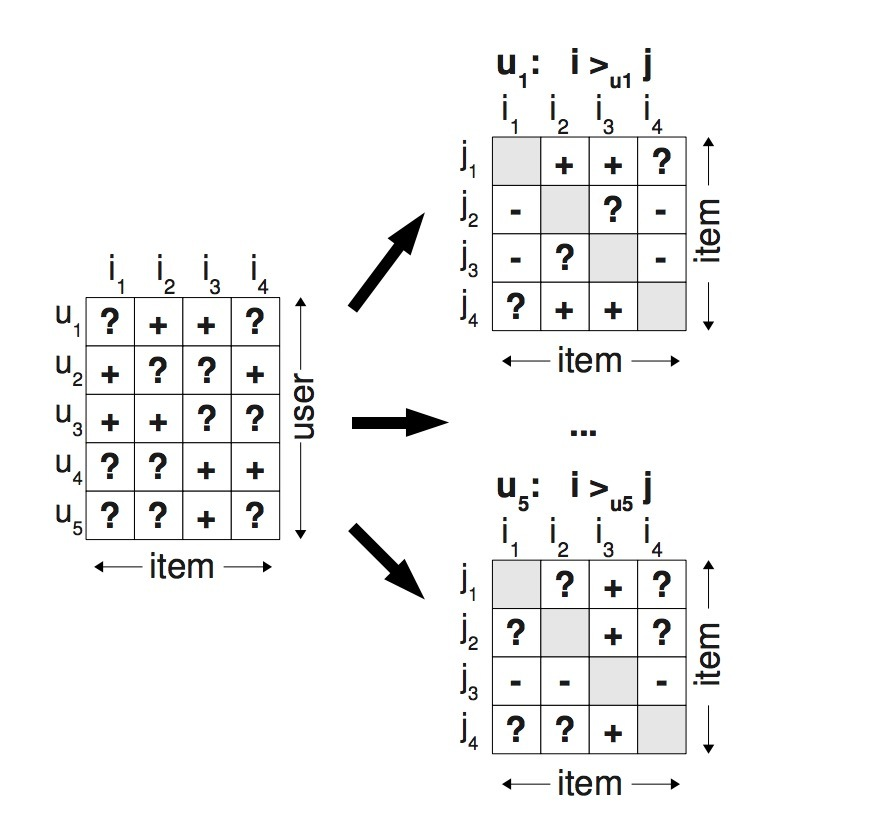$D_S := \lbrace (u, i, j) | i \in I_u^+ \wedge j \in I \backslash I_u^+ \rbrace$

$(u,i,j) \in D_S$的语义是，user $u$被假设成：喜欢i，胜过j。由于$>_u$是非对称的，负例会被隐式对待。

• 1.我们的训练数据同时包含了正负例pairs以及缺失值。介于两个未观察到的items间的缺失值是将来必须排序的item pairs。这意味着，从pairwise的角度看，训练数据$D_S$和测试数据是不相交的。
• 2.为排序的实际目标函数创建训练数据，例如：观察到$>_u$的子集$D_S$被用成训练数据。

# 4.BPR

## 4.1 BPR优化原则

$P(\Theta | >_u) \propto p(>_u | \Theta) p(\Theta)$

$\prod\limits_{u \in U} p(>_u | \Theta) = \prod\limits_{(u,i,j) \in U \times I \times I} p(i >_u j | \Theta)^{\delta((u,i,j) \in D_S)} \cdot(1-p(i >_u j | \Theta))^{\delta((u,j,i) \notin D_S}$

$\delta(b) := \begin{cases} 1 & \text{if b is true,} \\ 0 & \text{else} \end{cases}$

$\prod\limits_{u \in U} p(>_u | \Theta) = \prod\limits_{(u,i,j) \in D_S} p(i >_u j | \Theta)$

$p(i >_u j | \Theta) = \sigma( \hat{x}_{uij} (\Theta))$

• $\sigma$是logistic sigmoid：$\sigma(x) := \frac{1}{1+e^{-x}}$
• $\hat{x}_{uij}(\Theta)$是一个特定的关于模型参数向量$\Theta$的real-valued函数，它会捕获user u、item i、item j间的特殊关系。

$p(\Theta) \sim N(0, \sum_{\Theta})$

\begin{aligned} BPR-OPT &:= ln \ p(\Theta | >_u) \\ & = ln \ p(>_u | \Theta) p(\Theta) \\ & = ln \ \prod\limits_{(u,i,j) \in D_S} \sigma(\hat{x}_{uij}) p(\Theta) \\ & = \sum\limits_{(u,i,j) \in D_S} ln \ \sigma(\hat{x}_{uij}) + ln \ p(\Theta) \\ & = \sum\limits_{(u,i,j) \in D_S} ln \ \sigma(\hat{x}_{uij}) - \lambda_{\Theta} \|\Theta \|^2 \end{aligned}

### 4.1.1 AUC最优化分析

$AUC(u) := \frac{1}{ | I_u^+ | |I \backslash I_u^+ |} \sum\limits_{i \in I_u^+} \sum\limits_{j \in | I \backslash I_u^+|} \sigma(\hat{x}_{uij} > 0)$

$AUC := \frac{1}{|U|} \sum\limits_{u \in U} AUC(u)$

…(1)

$z_u = \frac{1} { | U | | I_u^+ | | I \backslash I_u^+|}$

$\sigma(x > 0) = H(x) := begin{cases} 1, & \text{ x > 0 } \\ 0, & \text{ else } end{cases}$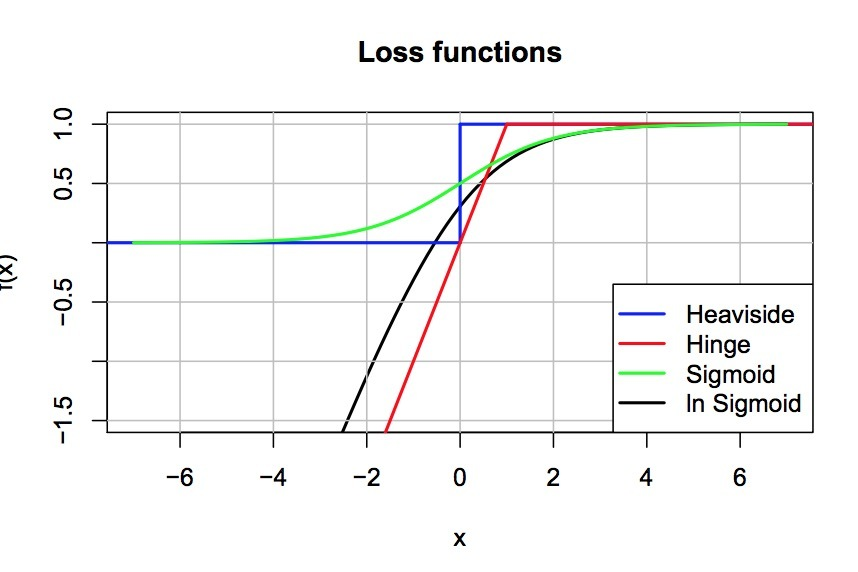## 4.2 BPR learning算法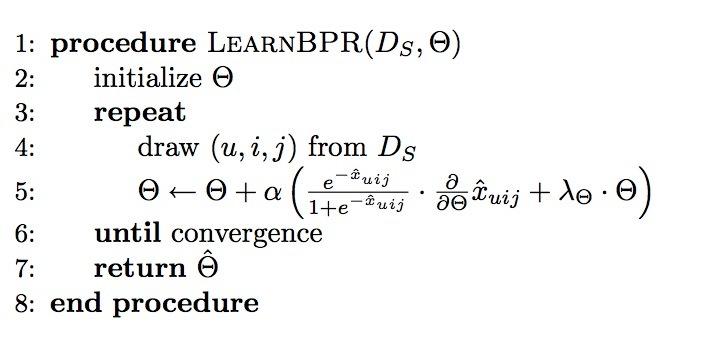\begin{aligned} \frac{\partial {BPR-OPT}}{\partial \Theta} & = \sum_{(u,i,j) \in D_S} \frac{\partial}{\partial \Theta} ln \sigma(\hat{x}_{uij}) - \lambda_{\Theta} \frac{\partial}{\partial \Theta} \| \Theta \|^2 \\ & \propto \sum\limits_{(u,i,j) \in D_S} \frac{-e^{-\hat{x}_{uij}}}{1+e^{-\hat{x}_{uij}}} \cdot \frac{\partial}{\partial \Theta} \hat{x}_{uij} - \lambda_{\Theta} \Theta \end{aligned}

$\theta \leftarrow \Theta - \alpha \frac{\partial BPR-OPT} {\partial \Theta}$

$\Theta \leftarrow \Theta + \alpha ( \frac{e^{-\hat{x}_{uij}}}{1+e^{\hat{x}_{uij}}} \cdot \frac{\partial}{\partial \Theta} \hat{x}_{uij} + \lambda_{\Theta} \Theta)$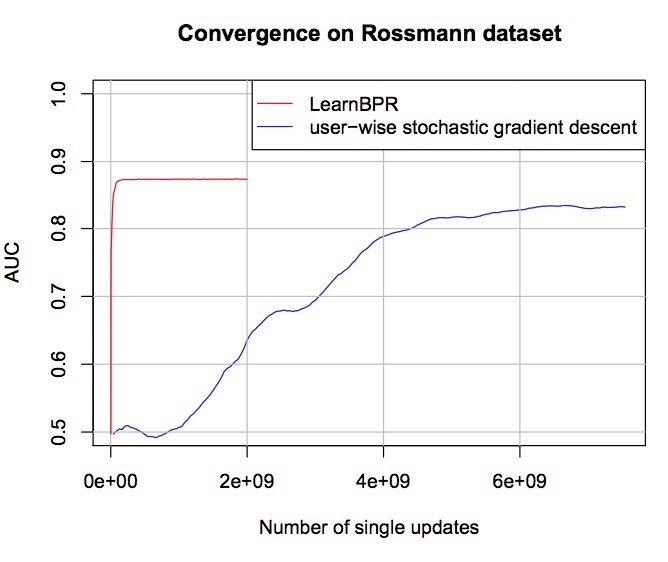## 4.3 使用BPR来学习模型

$\hat{x}_{uij} := \hat{x}_{ui} - \hat{x}_{uj}$

### 4.3.1 MF

$\hat{X} := W H^t$

$\hat{x}_{ui} = \langle w_u, h_i \rangle = \sum\limits_{f=1}^{k} w_{uf} \cdot h_{if}$

$\frac{\partial}{\partial \theta} \hat{x}_{uij} = \begin{cases} (h_{if} - h_{jf}) & \text{if \theta = w_{uf} } \\ w_{uf} & \text{if \theta = h_{if}} \\ -w_{uf} & \text{if \theta = h_{jf}} \\ 0 & \text{else} \end{cases}$

• $\lambda_W$对应用户特征W;

item features H有两个正则常数：

• $\lambda_{H^+}$被用于只在$h_{if}$上用于positive更新；
• $\lambda_{H^-}$用于在$h_{jf}$上的negative更新

$\hat{x}_{ui} = \sum\limits_{l \in I_u^+ \wedge l \neq i} c_{il}$

$c_{i,j}^{cosine} := \frac{|U_i^+ \cap U_j^+|} {\sqrt{ |U_i^+ | \cdot |U_j^+|}}$

$\frac{\partial}{\partial \Theta} \hat{x}_{uij} = \begin{cases} +1 & \text{if \theta \in \lbrace c_{il}, c_{li} \rbrace \wedge l \in I_u^+ \wedge l \neq i,} \\ -1 & \text{if \theta \in \lbrace c_{jl}, c_{lj} \rbrace \wedge l \in I_u^+ \wedge l \neq j,} \\ 0 & \text{else} \end{cases}$

# 5.与其它方法的关系

• WR-MF: Weighted Regularized Matrix Factorization
• MMMF: Maximum Margin Matrix Factorization

# 6.评估

## 6.1 datasets

• Rossmann dataset：来自在线电商。它包含了1w用户在4k items上的购买历史。总共有426612个购买记录。该任务是预测用户希望在下次购买的一个个性化items列表.
• Netflix DVD rental dataset: 该数据集包含了用户行为的打分，其中一个用户对电影提供了1-5星的显式评分。如果我们希望以隐式反馈的方式求解，我们可以移除rating。该任务是预测用户是否可能对一个电影进行评分。我们会为用户提供一个最可能打分的个性化排序列表。对于Netflix我们创建了一个subsample: 1w用户，5000 items，包含了565738个rating动作。我们会做子抽样：每个用户至少包含10个items，每个item至少有10个用户。

## 6.2 评估方法

$AUC = \frac{1}{|U|} \sum\limits_{u} \frac{1}{|E(u)|} \sum\limits_{(i,j) \in E(u)} \sigma(\hat{x}_{ui} > \hat{x}_{uj}$

…(2)

$E(u) := \lbrace (i,j) | (u,i) \in S_{test} \wedge (u,j) \notin (S_{test} \cup S_{train})rbrace$

…(2)

AUC越高表示效果越好。随机猜测法的AUC是0.5,最好为1。

## 6.3 结果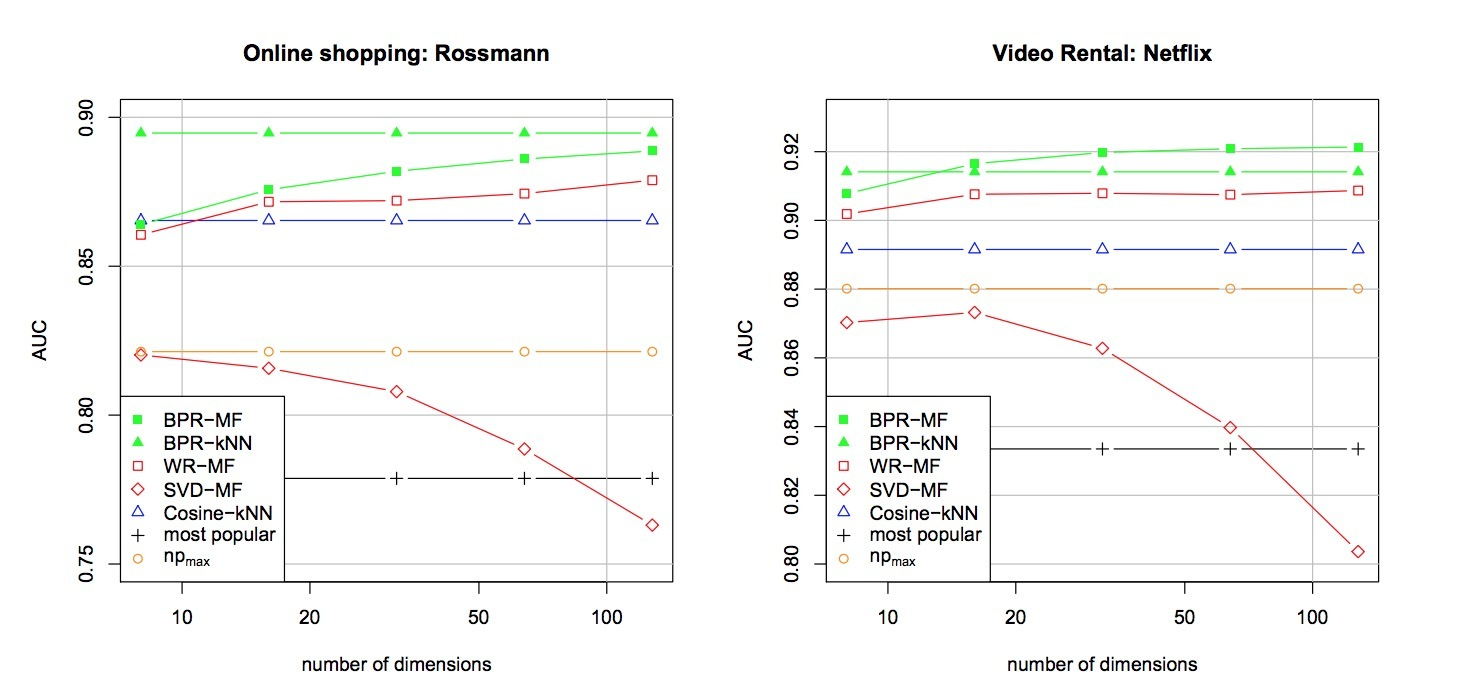# 参考

https://arxiv.org/pdf/1205.2618.pdf

# 1.介绍

LightLDA提出了一种花费更小的方法来解决这种大规模ML问题，在10台机器级别就能解决该问题。在三个级别上处理该问题：

• 1.以data-paralled 和 model-paralled方式实现分布式LDA inference：数据和模型会被分区（partitioned），接着跨机器进行流传输（streamed），以便在集群内更有效地利用内存和网络资源。
• 2.开发了一个Metropolis-Hastings sampler，对每个word/token，允许O(1)的采样时间，这可以在时间上产生一个高收敛率，可以击败当前state-of-art的samplers。
• 3.使用了一种不同的数据结构，利用海量语料，可以展示高频头部词，也可以展示低频长尾词，以不同的方式存储，有效利用资源，没有性能损失。

#

LDA实现分布variational- 和 sampling-based inference算法。LightLDA只关注sampling-based的方法。因为它可以产生非常稀疏的更新，使它们很适合设置很大的主题数K。

# 3.结构感知的模型并行(Structure-Aware Model Parallelism for LDA)• $\phi_{k} ~ Dirichlet(\beta)$: 为每个主题k抽取词分布$\phi_{k}$
• $\theta_d ~ Dirichlet(\alpha)$：为每个文档d抽取主题分布$theta_d$
• $n_d ~ Poisson(\gamma)$：对于每个文档d，抽取长度$n_d$（例如：它包含的tokens数目）
• 对于每个token $i \in { 1,2, \cdot, n_d }$：
• $z_{di} ~ Multinomial(\theta_{di})$: 抽取token的主题
• $w_{di} ~ Multinomial(\phi_{z_{di}})$：抽取token的词

$p(z_{di}=k\| rest) \propto \frac{(n_{kd}^{-di}+\alpha_k)(n_{kw}^{-di} + \beta_{w})}{n_k^{-di} + \hat{\beta}}$

…(1)• 1.workers不会移到下一个model slice，直到当前slice上相应的所有tokens都被抽样到，我们不需要对模型应用caching和eviction策略。

• 1.数据（the data）（包含tokens $w_{di}$和相应的topic indicators $z_{di}$）比模型(the model)更大（模型有万亿参数）
• 2.sampler收敛，模型会更加稀疏（这样可以减少网络通信），而数据的大小仍然是常数。

# 4.LDA的Fast Sampling算法

structure-aware model-parallelism的目的是：在小集群上，从十亿级别的文档中学到非常大的，上万亿参数的LDA模型；更进一步，bounded-asynchronous data-parallel schemes可使用parameter servers来减小网络同步和通信的开销。然而，这些还不能让大的LDA模型快速地进行训练；这激发了我们更大的贡献：一种新的LDA sampling算法，它比最新的算法（SparseLDA和AliasLDA）收敛地更快。为了解释我们的算法，先简单回顾下SparseLDA和AliasLDA的机制。

### SparseLDA

SparseLDA使用这样的观测：

• 1.大多数文档只有少量的主题
• 2.大多数词汇只参与少量的主题

$p(z_{di}=k\| rest) \propto \frac{\alpha_{k}\beta_w}{n_k^{-di}+\hat{\beta}} + \frac{n_{kd}^{-di}\beta_w}{n_k^{-di}+\hat{\beta}} + \frac{n_{kw}^{-di}(n_{kd}^{-di}+\alpha_{k)}{n_k^{-di}+\hat{\beta}}$

…(2)

### AliasLDA

AliasLDA提出了另一种的Gibbs sampling probability分解：

$p(z_{di}=k\| rest) \propto \frac{n_{kd}^{-di}(n_{kw}^{-di}+\beta_{w})}{n_{k}^{-di}+\hat{\beta}} + frac{\alpha_{k}(n_{kw}+\beta{w})}{n_k+\hat{\beta}}$

…(3)

## 4.1 Metropolis-Hastings sampling

• 1.从q(·)中抽取样本，比从p(·)中抽取样本更便宜
• 2.Markov chain可以快速混合（只需要一少部分step）

• 均匀分布Proposal（Uniform Distribution Proposal）: 假设我们选择了q(·)作为均匀分布。MH算法会提出：下一状态 t ~ Uni f(1,…,K) ，并接受$min(1, \frac{p(t)}{p(s)}$的概率的状态。很明显，从一个均匀分布中进行sampling是开销很小的，可以在O(1)时间内完成；然而，均匀分布是非稀疏的，因此与p(·)会相当远，它需要多步的MH来进行mix。

• 完全条件分布Proposal（Full Conditional Distribution Proposa）：我们可以选择p(·)作为proposal分布q(·)。MH算法提出了下一步t的概率为p(t)，接受$min{1, \frac{(p(t))p(s)}{p(s)p(t)}=1$；例如：算法会接受所有的proposals。从q(·)中抽样很明显开销与p(·)一样多，但mixing很很快，因为所有的proposals都被接受了。

## 4.2 因子分解（Factorization）的Cheap Proposals

$p(z_{di}=k\| rest) \propto \frac{(n_{kd}^{-di}+\alpha_k)(n_{kw}^{-di}+\beta_w)}{n_k^{-di}+\hat{beta}}$

…(4)

$p(z_{di}=k\| rest) \propto (n_{kd} + \alpha_{k}) x \frac{n_{kw}+\beta_w}{n_k + \hat{\beta}}$

…(5)

### Word-Proposal for Metropolis-Hastings

$p_w(k) \propto \frac{n_{kw}+\beta_{w}}{n_k+\hat{\beta}}$

…(6)

$min\{1, \frac{p(t)p_w(s)}{p(s)p_w(t)} \}$

…(7)

$\pi_w = \frac{(n_{td}^{-di}+\alpha_t)(n_tw^{-di}+\beta_w)(n_s^{-di}+\hat{\beta})(n_{sw}+\beta_w)(n_t+\hat{\beta})}{(n_{sd}^{-di}+\alpha_s)(n_{sw}^{-di}+\beta_w)(n_t^{-di}+\hat{\beta})(n_{tw}+\beta_w)(n_s+\hat{\beta})}$

…(8)

### Doc-Proposal for Metropolis Hastings

$p_d(k) \propto n_{kd} + \alpha_{k}$

…(9)

s->t状态转移的接受概率为：

$min(1, \frac{p(t)p_d(s)}{p(s)p_d(t)})$

…(10)

$\pi_d=\frac{(n_{td}^{-di}+\alpha_t)(n_{tw}^{-di}+\beta_w)(n_s^{-di}+\hat{\beta})(n_{sd}+\alpha_s)}{(n_{sd}^{-di}+\alpha_s)(n_{sw}^{-di}+\beta_w)(n_t^{-di}+\hat{\beta})(n_{td}+\alpha_t)}$

…(11)

$n_{kd} = \sum_{i=1}^{n_d}[z_{di}=k]$

…(12)

## 4.3 结合proposals提升Mixing

$p_c(k) \propto p_d(k) p_w(k)$

…(13)

# 5.对头部词(Power-Law Words)采用混合数据结构

• 1）在移除stop-words如果看，所有有意义的词汇的词汇几乎会超出32-bit integer的范围(2,147,483,647）；这在150亿文档和3w亿tokens，只保留300词频上的web-scale语料上测试时，会超过32-bit的限制。出于这个原因，我们选择使用32-bit的整数，而非64位。
• 2) 即使有数十亿的文档，大多数词的出现次数要少于K次（其中K是主题数，在我们的试验中达到了100w）。这意味着大多数行$n_k$，在word-topic table中是相当稀疏的，因此一个稀疏行表示（hash maps）将极大减小内存占用（memory footprint）。

• (1)大多数会访问我们的混合word-topic table中的dense arrays，这会保持高的throughput
• (2)word-topic中的大多数行仍是稀疏的hash tables，它可以让内存占用量合理保持较低水平

# 6.系统实现

Parameter Server和Data Placement 在基本水平上，一个parameter server(PS)会保存一个分布式共享内存接口，其中编程者可以从任何机器上访问内存，对于参数的物理位置不可知。本质上，PS扩展了在单个机器上的内存结构（如图6）；存储介质越接近CPU core，越具有较低的时延和较高的传输带宽，但有更少的容量（capacity）。在PS架构上，每个机器的RAM被分成两部分：对于客户端(client)使用的局部RAM，以及对于中心化参数存储的远程RAM（也称为：“server” part）。这样的硬件限制，以及由大的主题模型数据模型引入的需要条件，强烈地影响着我们运行Metropolis-Hastings算法的方式。

• (1)当前活动的工作数据集${ w_{di}, z_{di} }{d=1,i=1}^{D{S_n,n_d}}$
• (2)模型${ n_{kv} }{k=1,v=1}^{K,V_s}$需要抽样tokens的当前集合（使用Metropolis-Hastings sampler）。在抽样期间，我们更新token topic indicators $z{di}$，以及word-topic table。token-topic pairs（$w_{di}, z_{di}$）位于本地worker上，不会有网络通信，而word-topic table则被存储在PS中，因而需要一个后台线程来进行有效通信。

Token 和 Topic Indicator Storage 作为data-parallel执行的一部分，每个worker机器会在本地磁盘上存储语料的某个shard。对于web-scale级别的语料，每个shard仍会很大——如果没有许多T，也会有数百G——它不会将整个shard加载进内存中。这样，我们进一步将每个数据的shard分割成数据块（data blocks），并将这些块同时流式化进内存中（见图1左）。根据数据结构，我们故意将tokens $w_{di}$和它们的topic indicators $z_{di}$进行side-by-side放置，作为一个$(w_{di}, z_{di})$ pair的向量(vector)，而非两个独立的tokens和topic indicators数组。我们这么做是为了提升数据的locality和CPU cache更有效：无论何时当我们访问一个token $w_{di}$时，我们总是需要访问它的topic indicator $z_{di}$，vector-of-pairs的设计方式可以直接提升locality。这种设计的一个缺点是：额外的磁盘I/O，每次读/写 tokens $w_{di}$一个数据shard到磁盘中时会swap out。然而，磁盘I/O总是通过对读/写进行pipeline的方式进行masked，当sampler正处理当前shard时会在后台完成。

• 1.在计算一个数据块或一个模型切片时，一个worker的CPU core需要等待下一个数据块/模型切片从磁盘/网络被加载。我们通过pipelinging（如图7所示）消除了该I/O延迟，尽管我们注意到：执行pipelining需要很仔细的参数配置（samplers的throughout，数据块的size，模型切片的size等）
• 2.为了阻止数据加载在跨模型切片时的imbalance，我们通过对词汇表通过词频进行排序来生成模型切片，接着对词汇进行shuffing。这种方式下，每个切片会同时包含热词（hot words）和长尾词（long tail words），来改善加载的平衡。
• 3.为了消除数据传输的不必要性，当生成数据块时，我们对token-topic pair $w_{di}, z_{di}$根据$w_{di}$在重排后(shuffled)的词汇表中的位置进行排序，确保属于相同的模型切片的所有tokens在数据块上实际是连续的（见图1）。这种排序只需要执行一次，在数据处理平台如Hadoop上会很快（对比于LDA sampling time）。我们认为：比起PLDA+中的”word bundle”，这种方式更有效，PLDA+会用一个倒排索引来避免数据传输，但会带来两倍的内存开销。

# 7.试验数据

• (1)在core数目和机器数目上，我们的分布式实现有接近线性的可扩展性
• (2)比起state-of-art的SparseLDA和AliasLDA samplers，我们的分布式实现有接近线性的可扩展性（在单线程设置中测量得到）
• (3)最重要的是，LightLDA可以允许很大的数据size和模型size，只需要8台左右机器即可。

# 参考

LightLDA: Big Topic Models on Modest Compute Clusters